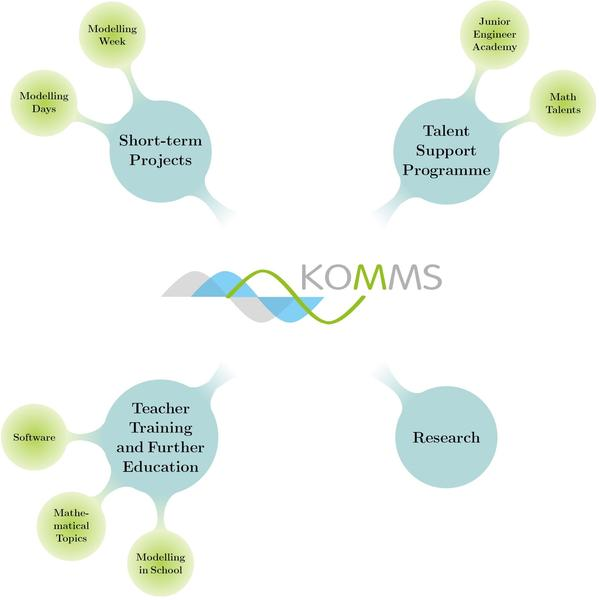# Competence Centre for Mathematical Modelling in STEM-Projects in Schools (KOMMS)What is KOMMS?

KOMMS, the competence centre for mathematical modelling in STEM (science, technology, engineering and mathematics) projects in schools, was founded by the mathematics faculty at the University of Kaiserslautern with the aim of consolidating and improving the teaching of mathematical modelling in schools.  This is achieved through the provision and coordination of school projects, ranging from single day to week-long projects, along with the support and development of talented individuals through the talent support programme. The school projects and support programmes are underpinned by research into didactical methods along with teacher training, ensuring the selection of age-appropriate modelling projects and the sustainability of the project.

KOMMS supports the state of Rhineland-Palatinate with respect to future developments of questions regarding the education of STEM-subjects.

Since 2015, the modelling days and weeks are funded by the European Social Fund (ESF) within the framework of the project Development of Mathematical Modelling in STEM-subjects in Schools (German: Schulentwicklung für Mathematische Modellierung in MINT-Fächern, SchuMaMoMINT).What do we mean by “mathematical modelling”?

There exist many different definitions of what mathematical modelling is what it and encompasses. In a broad sense, mathematical modelling can be defined as using mathematical terms and methods, be it algebra, graphs, tables, probability, etc. to represent a problem, be it a system or a process. However, for our purpose, we wish to restrict this definition to:

Mathematical modelling is using mathematical terms and methods to represent an open-ended and unstructured problem based on a real life process or system.

In other words, in posing the problem to be studied, the following three statements must apply:

1. the posed problem has some relation to a process or system found in real life;
2. a method for approaching the problem is not directly obvious to the student and thus challenges that student;
3. there exists no one correct method for approaching the problem or obtaining a “solution”.

Why do we think that including mathematical modelling within schools is important?

Engaging in such types of open ended and unstructured problems has been shown, in a number of separate research studies, to be highly beneficial for students and lead to very positive results. In particular, undertaking such mathematical modelling problems as defined above, can:

extend students’ mathematical competence by engaging them in mathematical thinking and extending their mathematical understanding beyond learning by rote;

• result in improved mathematical ability;
• develop problem solving skills, determination, flexibility, perseverance, inquisitiveness;
• result in increased self-confidence;
• result in an increased ability to self-critique and self-monitor;
• develop and encourage independent thinking;
• develop competence in related scientific subjects (physics, computer science, etc.);
• make mathematics more relevant and interesting.

Furthermore, these type of problems lend themselves to students working in teams, which further develops:

• teamworking skills;
• communication skills;
• presentation skills.